# multirotorFlightTrajectory

Multirotor UAV trajectory

## Description

The `multirotorFlightTrajectory` object stores a multirotor UAV trajectory created by using piecewise 9th degree polynomial to interpolate linear positions and the `cubicpolytraj` function to interpolate yaw angles between specified waypoints.

## Creation

### Syntax

``T = multirotorFlightTrajectory(wpts,vels,accs,jerks,snaps,yaws,toas)``
``T = parse(parser,mission)``

### Description

example

````T = multirotorFlightTrajectory(wpts,vels,accs,jerks,snaps,yaws,toas)` creates a `multirotorFlightTrajectory` object, `T` using the specified waypoints `wpts`, velocities `vels`, accelerations `accs`, jerks `jerks`, snaps `snaps`, yaws `yaws`, and times of arrival `toas`. The input arguments set the `Waypoints`, `Velocities`, `Accelerations`, `Jerks`, `Snaps`, `Yaws`, and `TimeOfArrival` properties, respectively.```
````T = parse(parser,mission)` parses a UAV mission `mission` using a multirotor mission parser `parser` to create a `multirotorFlightTrajectory` object.```

## Properties

expand all

Waypoints, specified as an N-by-3 matrix in the local north-east-down (NED) reference frame, in meters. N is the total number of waypoints, and each row contains the xyz-coordinates of a waypoint, [X Y Z].

Example: `[2 1 3; 3 2 4]`

Desired velocities, specified as an N-by-3 matrix in the local north-east-down (NED) reference frame, in meters per second. N is the total number of waypoints, and each row is the desired velocity of the UAV at the corresponding waypoint, [dX dY dZ].

Example: `[2 1 3; 3 2 4]`

Desired accelerations, specified as an N-by-3 matrix in the local north-east-down (NED) reference frame, in m/s2. N is the total number of waypoints, and each row is the desired acceleration at the corresponding waypoint, [d2X d2Y d2Z].

Example: `[2 1 3; 3 2 4]`

Desired jerks, specified as an N-by-3 matrix in the local north-east-down (NED) reference frame, in m/s3. N is the total number of waypoints, and each row is the desired jerk at the corresponding waypoint, [d3X d3Y d3Z].

Example: `[2 1 3; 3 2 4]`

Desired snaps, specified as an N-by-3 matrix in the local north-east-down (NED) reference frame, in m/s4. N is the total number of waypoints, and each row is the desired snap at the corresponding waypoint, [d4X d4Y d4Z].

Example: `[2 1 3; 3 2 4]`

Desired yaw angles, specified as an N-element vector in the local north-east-down (NED) reference frame, in degrees. N is the total number of waypoints, and each row is the desired yaw at the corresponding waypoint.

Example: `[20;32;0;-20]`

Times of arrival, specified as an N-element column vector, in seconds. N is the total number of waypoints, and each row is the time of arrival at the corresponding waypoint.

The first and last elements of `TimeOfArrival` set the `StartTime` and `EndTime` properties, respectively.

Example: `[2; 3; 5; 6]`

Start time of the trajectory, stored as a nonnegative numeric scalar, in seconds. The value of `StartTime` is the same as the value of the first element of the `TimeOfArrival` property.

End time of the trajectory, stored as a nonnegative numeric scalar, in seconds. The value of `EndTime` is the same as the value of the last element of the `TimeOfArrival` property.

## Object Functions

 `copy` Copy flight trajectory `show` Visualize the UAV trajectory `query` Get UAV motion vectors at timestamps

## Examples

collapse all

Create a UAV mission by using the flight plan stored in a `.plan` file and show the mission.

```mission = uavMission(PlanFile="flight.plan"); show(mission);```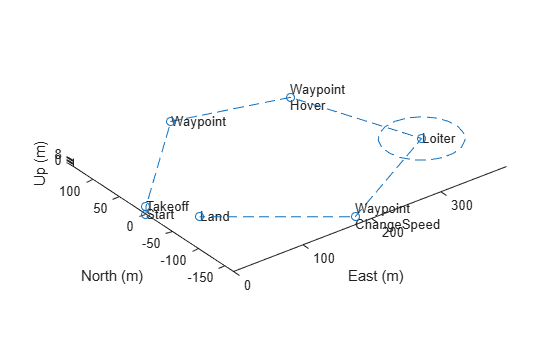Create parsers for a multirotor UAV and a fixed-wing UAV.

```mrmParser = multirotorMissionParser(TransitionRadius=2,TakeoffSpeed=2); fwmParser = fixedwingMissionParser(TransitionRadius=15,TakeoffPitch=10);```

Generate one flight trajectory using each parser.

```mrmTraj = parse(mrmParser,mission); fwmTraj = parse(fwmParser,mission);```

Visualize the mission and the flight trajectories separately.

```figure subplot(1,2,1) show(mrmTraj); title("Multirotor Trajectory") axis square subplot(1,2,2) show(fwmTraj); title("Fixed-Wing Trajectory") axis square```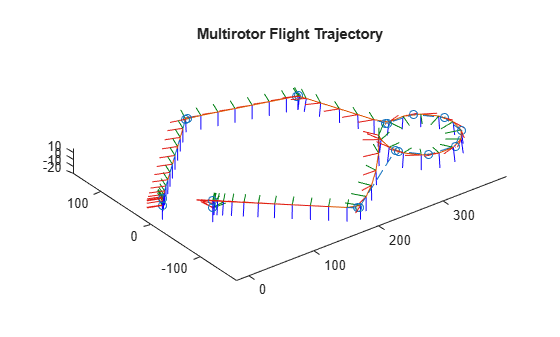Plot the mission and flight trajectories overlapping.

```figure show(mission); hold on show(mrmTraj); hold off title("Mission Using Multirotor Trajectory") axis square```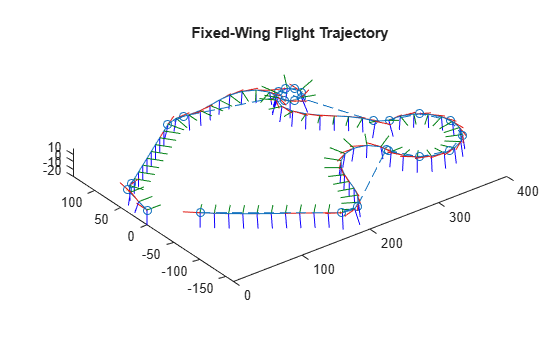```show(mission); hold on show(fwmTraj); hold off title("Mission Using Fixed-Wing Trajectory") axis square```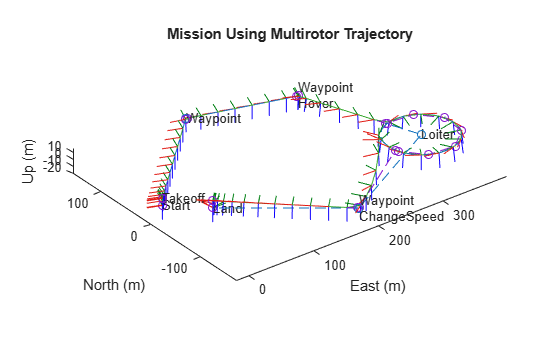Create a set of waypoints for both the multirotor and the fixed-wing UAV to follow.

```wpts = [0 0 0; 2 2 -2; 10 10 -3; 12 12 -6]; numwpts = size(wpts);```

Specify additional trajectory information, such as desired velocities, accelerations, jerks, snaps, and yaws, as well as start time, an end time, and times of arrival.

```vels = 2*ones(numwpts); accs = ones(numwpts); jerks = zeros(numwpts); snaps = zeros(numwpts); yaws = zeros(1,numwpts(1)); starttime = 0; endtime = 8; toas = linspace(starttime,endtime,numwpts(1));```

Use the trajectory information to create the flight trajectories for the multirotor and the fixed-wing UAVs. Query and display the trajectories.

```mrft = multirotorFlightTrajectory(wpts,vels,accs,jerks,snaps,yaws,toas); fwft = fixedwingFlightTrajectory(wpts,vels,toas); query(mrft,1:4)```
```ans = 4×16 1.6184 1.6184 0.7520 -0.0243 -0.0243 -2.8758 -4.6045 -4.6045 -9.1669 0.9863 -0.1179 0.1147 -0.0137 0.0732 -0.0732 -0.0000 1.0236 1.0236 -2.7807 0.5482 0.5482 -0.6198 3.9704 3.9704 8.6424 0.7429 0.5559 -0.2987 -0.2235 -1.3257 1.3257 0 2.7277 2.7277 -1.2947 2.4069 2.4069 2.1026 1.7442 1.7442 -1.3857 0.9941 0.0770 -0.0761 -0.0059 0.1950 -0.1950 0 6.4028 6.4028 -2.0972 4.4609 4.4609 -3.8447 -1.1875 -1.1875 -1.1875 0.9971 -0.0537 0.0534 -0.0029 -1.2364 1.2364 -0.0000 ```
`query(fwft,1:4)`
```ans = 4×16 0.9453 0.9453 -0.3203 0.2422 0.2422 -1.8672 -0.7031 -0.7031 -1.5469 0.7098 -0.2450 0.5914 0.2940 -0.4683 0.4683 0.0000 1.1875 1.1875 -2.1875 0.5938 0.5938 -1.0938 1.4063 1.4062 3.0938 0.8287 -0.1692 0.4085 0.3432 1.7750 -1.7750 0 2.7813 2.7812 -1.6055 2.6563 2.6562 0.4414 1.6875 1.6875 -4.0078 0.9223 0.0224 -0.0540 0.3820 -0.7962 0.7962 -0.0000 6.0000 6.0000 -2.5000 3.5000 3.5000 -1.5625 -0.0000 -0.0000 0.0000 0.9131 -0.0583 0.1407 0.3782 -0.0000 -0.0000 0.0000 ```

Visualize both the multirotor flight trajectory and the fixed-wing flight trajectory.

```ax = show(mrft,NumSamples=200); title("Multirotor Flight Trajectory") view([0 0])```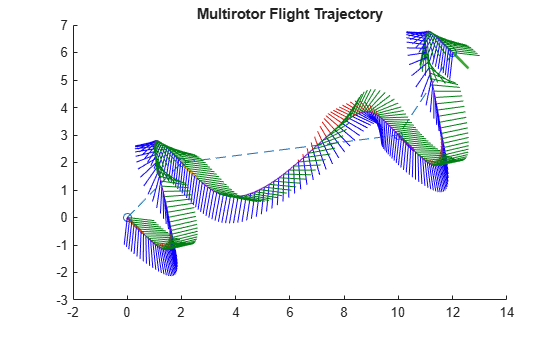```show(fwft,NumSamples=50); title("Fixed-Wing UAV Flight Trajectory") view([0 0])```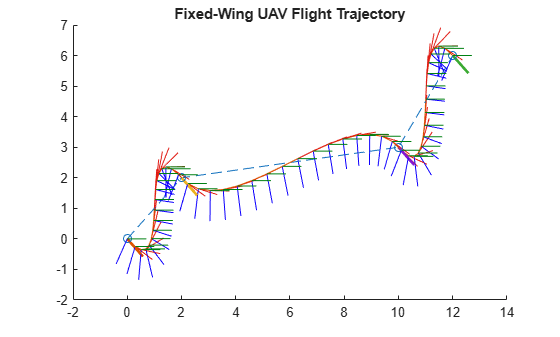## Version History

Introduced in R2022b